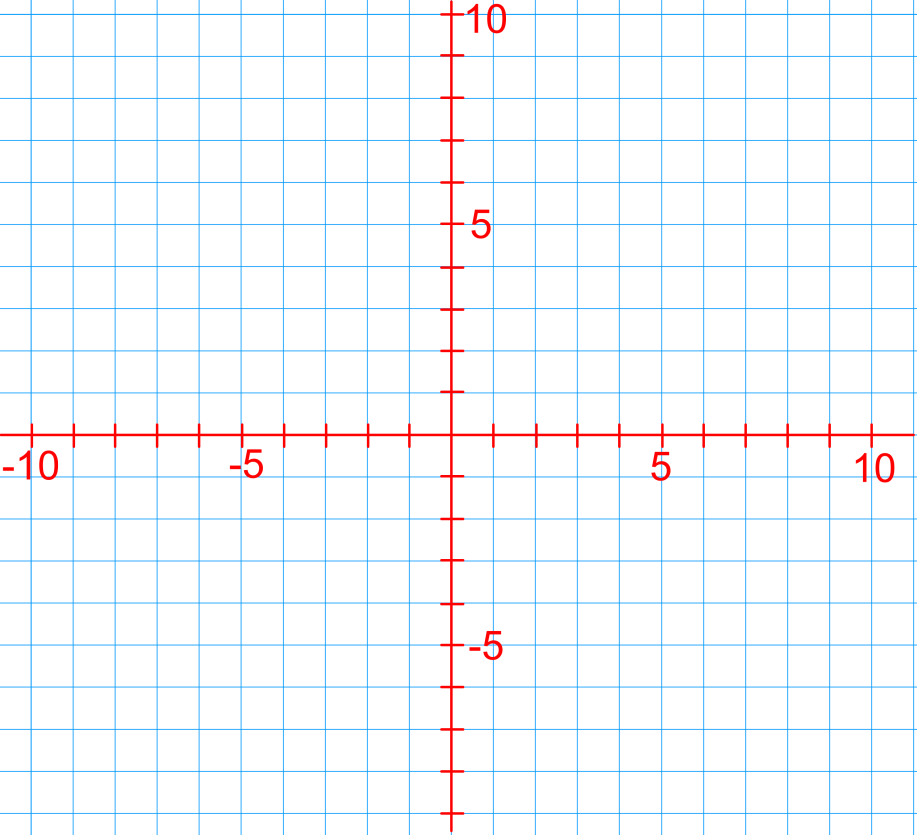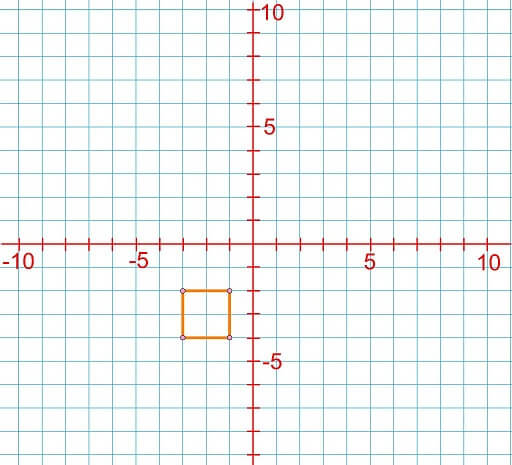# Draw on coordinate planes

### Draw on coordinate planes

In previous section, we learned how to label and plot coordinates on given grids. Astronomers often use grids and coordinates to determine the distance between bodies, such as planets, in space. In this section, we will draw figures on coordinate grids by connecting points on grids. The points will be connected in alphabetical order using straight lines. Finally, we will write instructions for how to draw these figures.

#### Lessons

• 1.
On a coordinate grid, draw a square in the following quadrants and write the instructions for drawing it. In your instructions, mention the (x,y) coordinates and labels (A, B, C, etc.) of the points.

i) Quadrant I

ii) Quadrant II

iii) Quadrant III• 2.
Label each vertex of the shape and identify the coordinates.